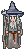# Find date of saturday of the week with current date in php

i wana get date of saturday of current week with date of current day,how its possible,im googling but nothing found,

I think what you’re looking for is strtotime()1 Like

Assuming that you have the original date in \$dt and want Saturday’s date in \$dt1 then we can do:

``````\$dt1 = DateTime::createFromFormat(('Y-m-d', date('Y-m-',\$dt).(6+date('j',\$dt)-date('w',\$dt)));
``````
1 Like

thanks but what is the format of \$dt?

when i run this code `\$dt=date("d"); \$dt1 = DateTime::createFromFormat(('Y-m-d',date('Y-m-',\$dt).(6+date('j',\$dt)-date('w',\$dt))); echo \$dt1;`

i get this error ` Parse error: syntax error, unexpected ',' in ...`
this error belongs to line of `\$dt1 = DateTime::createFromFormat(('Y-m-d', date('Y-m-',\$dt).(6+date('j',\$dt)-date('w',\$dt)));`

i think i maked a mistake,the format of \$dt?

\$dt would be a date object.

1 Like

i have same parse error,Parse error: syntax error, unexpected ‘,’ in …

`\$dt = new DateTime(‘02/31/2011’);

\$dt1 = DateTime::createFromFormat((‘Y-m-d’,date(‘Y-m-’,\$dt).(6+date(‘j’,\$dt)-date(‘w’,\$dt)));
echo \$dt1;`

i maked some change on this code and worked awesome, @felgall you are genius, works perfectly

thanks to you mr @Gandalf i used strtotime for this success,

1 Like

This topic was automatically closed 91 days after the last reply. New replies are no longer allowed.